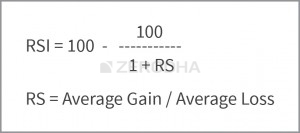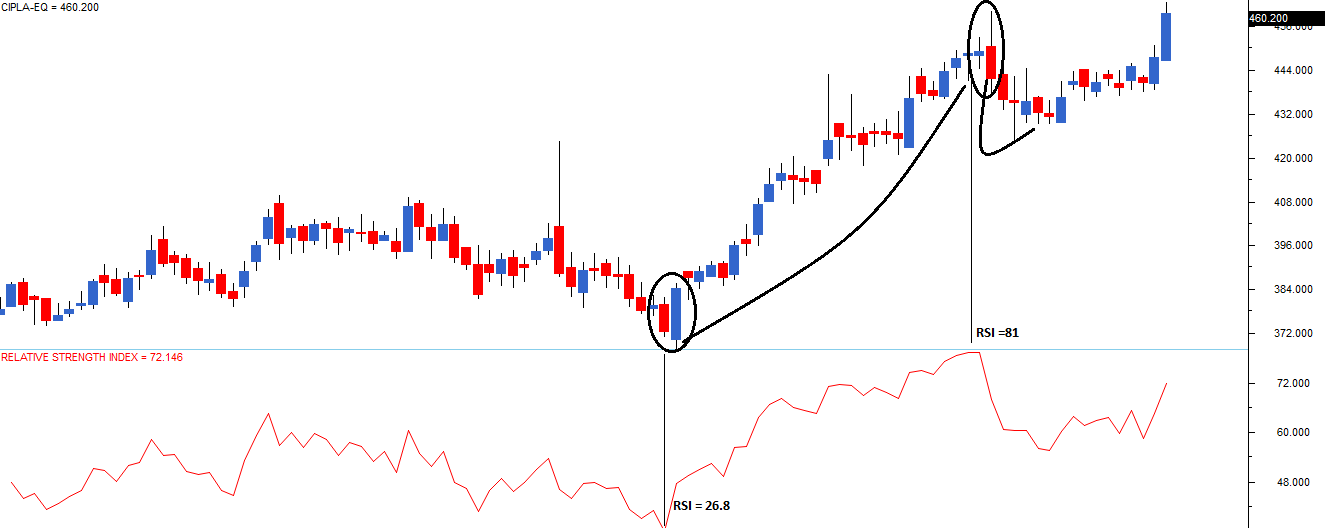## Relative Strength Index

Relative strength Index or just RSI, is a very popular indicator developed by J.Welles Wilder. RSI is a leading momentum indicator which helps in identifying a trend reversal. RSI indicator oscillates between 0 and 100, and based on the latest indicator reading, the expectations on the markets are set.
The term “Relative Strength Index” can be a bit misleading as it does not compare the relative strength of two securities, but instead shows the internal strength of the security. RSI is the most popular leading indicator, which gives out strongest signals during the periods of sideways and non trending ranges.
The formula to calculate the RSI is as follows:Let us understand this indicator with the help of the following example:
Assume the stock is trading at 99 on day 0, with this in perspective; consider the following data points:
Sl NoClosing PricePoints GainPoints Lost
0110010
0210220
0310530
0410720
0510304
0610003
079901
089702
0910030
1010550
1110720
1211030
1311440
1411840
Total2910
In the above table, points gained/lost denote the number of points gained/lost with respect to the previous day close. For example if today’s close is 104 and yesterday’s close was 100, points gained would be 4 and points lost would be 0. Similarly, if today’s close was 104 and previous day’s close was 107, the points gained would be 0 and points lost would be 3. Please note that, the loses are computed as positive values.
We have used 14 data points for the calculation, which is the default period setting in the charting software. This is also called the ‘look-back period’. If you are analyzing hourly charts the default period is 14 hours, and if you are analyzing daily charts, the default period is 14 days.
The first step is to calculate ‘RS’ also called the RSI factor. RS as you can see in the formula, is the ratio of average points gained by the average points lost.
Average Points Gained = 29/14
= 2.07
Average Points Lost = 10/14
= 0.714
RS = 2.07/0.714
= 2.8991
Plugging in the value of RS in RSI formula,
= 100 – [100/ (1+2.8991)]
= 100 – [100/3.8991]
= 100 – 25.6469
RSI = 74.3531
As you can see RSI calculation is fairly simple. The objective of using RSI is to help the trader identify over sold and overbought price areas. Overbought implies that the positive momentum in the stock is so high that it may not be sustainable for long and hence there could be a correction. Likewise, an oversold position indicates that the negative momentum is high leading to a possible reversal.
Take a look at the chart of Cipla Ltd, you will find a lot of interesting developments:To begin with, the red line below the price chart indicates the 14 period RSI. If you notice the RSI’s scale you will realize its upper bound to 100, and lower bound to 0. However 100 and 0 are not visible in the chart.
When the RSI reading is between 30 and 0, the security is supposed to be oversold and ready for an upward correction. When the security reading is between 70 and 100, the security is supposed to be heavily bought and is ready for a downward correction.
The first vertical line marked from left shows a level where RSI is below 30, in fact RSI is 26.8. Hence RSI suggests that the stock is oversold. In this particular example, the RSI value of 26.8, also coincides with a bullish engulfing pattern. This gives the trader a double confirmation to go long! Needless to say, both volumes and S&R should also confirm to this.
The second vertical line, points to a level where the RSI turns 81, a value which is considered overbought. Hence, if not for looking at shorting opportunities, the trader should be careful in his decision to buy the stock. Again, if you notice the candles, they form a bearish engulfing pattern. So a bearish engulfing pattern, backed by an RSI of 81 is a sign to short the stock. What follows this is a quick and a short correction in the stock.
The example that I have shown here is quite nice, meaning both the candlestick pattern and RSI perfectly align to confirm the occurrence of the same event. This may not always be true. This leads us to another interesting way to interpret RSI. Imagine the following two scenarios:
Scenario 1) A stock which is in a continuous uptrend (remember the uptrend can last from few days to few years) the RSI will remain stuck in the overbought region for a long time, and this is because the RSI is upper bound to 100. It cannot go beyond 100. Invariably the trader would be looking at shorting opportunities but the stock on the other hand will be in a different orbit. Example – Eicher motors Limited, the stock has generate a return of close to 100% year on year.
Scenario 2) A stock which is in a continuous downtrend the RSI will be stuck in the oversold region since the RSI is lower bound to 0. It cannot go beyond 0. In this case as well the trader will be looking at buying opportunities but the stock will be going down lower. Example – Suzlon Energy, the stock has generated a return of negative 34% year on year.
This leads us to interpret RSI in many different ways besides the classical interpretation (which we discussed earlier)
1. If the RSI is fixed in an overbought region for a prolonged period, look for buying opportunities instead of shorting. The RSI stays in the overbought region for a prolonged period because of an excess positive momentum
2. If the RSI is fixed in an oversold region for a prolonged period, look for selling opportunities rather than buying. RSI stays in the oversold region for a prolonged period because of an excess negative momentum
3. If the RSI value starts moving away from the oversold value after a prolonged period, look for buying opportunities. For example, the RSI moves above 30 after a long time may mean that the stock may have bottomed out, hence a case of going long.
4. If the RSI value starts moving away from the overbought value after a prolonged period, look for selling opportunities. For example, RSI moving below 70 after a long time. This means the stock may have topped out, hence a case for shorting

## 14.2 – One last note

None of the parameters used while analyzing RSI should be treated with rigidity. For example, J.Welles Wilder opted to use a look back period of 14 days simply because that was the value which gave the best results considering the market conditions in 1978 (which is when RSI was introduced to the world). You may choose to use 5,10,20, or even 100 days look back period if you wish too. In fact this is how you develop your edge as a trader. You need to analyze what works for you and adopt the same. Please note, fewer the days you use to calculate the RSI, the more volatile the indicator would be.
Also, J.Welles Wilder decided to use 0-30 level to indicate oversold regions and 70-100 level to indicate overbought region. Again this is not set in stone, you can arrive at you own combination.
I personally prefer to use 0-20 level and 80-100 level to identify oversold and overbought regions respectively. I use this along with the classical 14 day look back period.
Of course, I urge you to explore parameters that work for you. In fact this is how you would eventually develop as a successful trader.
Finally, do remember RSI is not used often as a standalone indicator by  traders, it is used along with other candlestick patterns and indicators to study the market.

### Key takeaways from this chapter

1. Indicators are independent trading systems developed, and introduced by successful traders
2. Indicators are leading or lagging. Leading indicators signals the possible occurrence of an event. Lagging indicators on the other hand confirms an ongoing trend
3. RSI is a momentum oscillator which oscillates between 0 and 100 level
4. A value between 0 and 30 is considered oversold, hence the trader should look at buying opportunities
5. A value between 70 and 100 is considered overbought, hence the trader should look at selling opportunities
6. If the RSI value is fixed in a region for a prolonged period, it indicates excess momentum and hence instead of taking a reversed position, the trader can consider initiating a trade in the same direction.